# CBSE Sample Papers for Class 12 Economics Paper 2

CBSE Sample Papers for Class 12 Economics Paper 2 are part of CBSE Sample Papers for Class 12 Economics. Here we have given CBSE Sample Papers for Class 12 Economics Paper 2.

## CBSE Sample Papers for Class 12 Economics Paper 2

 Board CBSE Class XII Subject Economics Sample Paper Set Paper 2 Category CBSE Sample Papers

Students who are going to appear for CBSE Class 12 Examinations are advised to practice the CBSE sample papers given here which is designed as per the latest Syllabus and marking scheme as prescribed by the CBSE is given here. Paper 2 of Solved CBSE Sample Paper for Class 12 Economics is given below with free PDF download solutions.

Time : 3 hrs
M.M.: 80

General Instructions

• All questions in both the sections A and B are compulsory. However, there is internal choice in questions of 3,4 and 6 marks.
• Question Nos. 1-4 and 13-16 are very short answer type questions, carrying 1 mark each.
They are required to be answered in one sentence each.
• Question Nos. 5-6 and 17-18 are short answer I type questions, carrying 3 marks each.
Answers to them should not normally exceed 60 words each.
• Question Nos. 7-9 and 19-21 are short answer II type questions, carrying 4 marks each.
Answers to them should not normally exceed 70 words each.
• Question Nos. 10-12 and 22-24 are long answer type questions, carrying 6 marks each.
Answers to them should not normally exceed 100 words each.
• Answers should be brief and to the point and the above word limit be adhered to as far as possible.

Section – A

Question 1.
State the fundamental problem of an economy that resulted in the emergence of central economic problem.

Question 2.
Average revenue of a monopolist firm is
(a) always more than the marginal revenue
(b) always less than the marginal revenue
(c) equal to marginal revenue
(d) None of these

Question 3.
Total Revenue (TR) – Total Variable Cost (TVC) is equal to
(a) gross profit
(b) net profit
(c) normal profit
(d) abnormal profit

Question 4.
How is total variable cost derived from marginal cost?

Question 5.
Suppose there are 20 consumers for a good and they have identical demand functions. d(p) = 50 – 6p for any price less than or equal to $$\frac { 20 }{ 6 }$$ and d(p) = 0 at any price greater than $$\frac { 10 }{ 3 }$$.
What is the market demand function?
Or
Consider the demand curve d(p)= 10 – 3p. What is the elasticity at price $$\frac { 5 }{ 3 }$$ ?

Question 6.
What will be the impact on India’s PPC, if latest technology is imported from abroad?

Question 7.
Draw a diagram indicating the following situations:
(i) $$XY>\frac { { P }_{ X } }{ { P }_{ Y } }$$
(ii) $$XY=\frac { { P }_{ X } }{ { P }_{ Y } }$$
(iii) $$XY<\frac { { P }_{ X } }{ { P }_{ Y } }$$
Where, XY = Marginal Rate of Substitution of Good x for Good y
Px = Price of Good X, PY = Price of Good y
Which of the three indicates highest level of consumer’s satisfaction?
Or
A consumer consumes only two goods X and Y. State and explain the conditions of consumer’s equilibrium with the help of utility analysis.

Question 8.
Explain the ‘law of variable proportions’. Use schedule.

Question 9.
What do you understand by oligopoly market? Explain any three of its features.

Question 10.
Define demand. Explain the factors influencing individual household demand for a commodity in the market.

Question 11.
(i) Can Marginal Revenue (MR) be negative? Explain your answer with the help of example.
(ii) Total Revenue (TR) of a firm, dealing in a particular commodity, initially was ₹ 15,000. It reduces to ₹ 13,500. Also, AR increases from ₹150 to ₹ 270. Find the change in market demand for that commodity.
Or
State whether the following statements are true or false. Give reasons for your answer.
(i) When Marginal Revenue (MR) is constant and not equal to zero, then Total Revenue (TR) will also be constant.
(ii) As soon as Marginal Cost (MC) starts rising, Average Variable Cost (AVC) also starts rising.
(iii) Total Product (TP) always increases whether there is increasing returns or diminishing returns to a factor.

Question 12.
Explain profit and loss situation under perfect competition, during the short-run. Which economic value is affected if the firm is incurring abormal losses?

Section – B

Question 13.
What are the sources of borrowings for government other than the general public?

Question 14.
The currency notes do not carry as much value as denominated on it. Do you agree?

Question 15.
What do you mean by public goods?

Question 16.
The difference between narrow money and broad money is
(a) coins and currency
(b) only currency
(c) saving deposits of banks
(d) time deposits with banks

Question 17.
The saving function of an economy is S = -200 + 0.25F. The economy is in equilibrium when income is equal to ₹ 2,000. Calculate the following :
(i) Investment expenditure at equilibrium level of income
(ii) Autonomous consumption
Or
It is given that MPC is 1/3rd of MPS, while consumption at zero level of income is given as ₹ 100 crore. Derive the consumption and savings functions.

Question 18.
Inflationary gap and deflationary gap both are caused due to the displacement of aggregate demand from the full employment equilibrium level and both are harmful for a country. In spite of these similarities, there exist many differences between the two. Explain any three of those differences.

Question 19.
How will you treat the following while estimating national income of India?
(i) Dividend received by an Indian from his investment in shares of a foreign company.
(ii) Money received by a family in India from relatives working abroad.
(iii) Interest received on loans given to a friend for purchasing a car.
(iv) Dividend received by a foreigner from investment shares of an Indian company.

Question 20.
Giving a numerical example, explain the process of money creation by the commercial banks.
Or
Government of India has launched ‘Jan-Dhan Yojana’ aimed at every household to have at least one bank account due to which credit creation capacity of banks have seen expansion manifolds. Comment.

Question 21.
Explain the role of government budget in fighting inflationary and deflationary tendencies.

Question 22.
From the following information, calculate Gross National Product at Market Price (GNPMP)by
(i) Income method
(ii) Expenditure method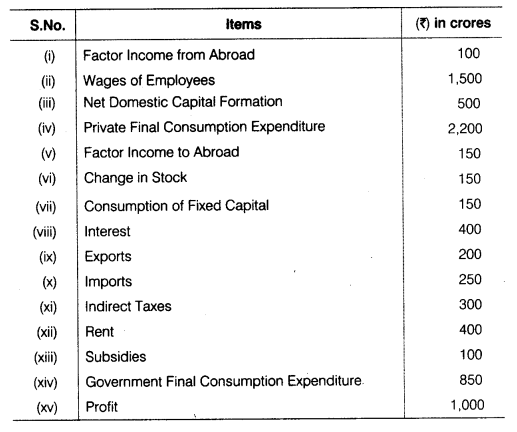Or
Explain briefly the steps taken in estimating national income by the value added method. Also, explain any two precautions required to be taken in this method

Question 23.
What do you mean by Multiplier (K)? Explain the relationship between Marginal Propensity to Consume (MPC) and Multiplier (K).

24.
Explain the three major functions of foreign exchange market.

Section – A

It is the problem of scarcity of resources in relation to human needs or desires.

(a) Always more than marginal revenue

(a) Gross profit

Total variable cost is the sum total of marginal cost, i.e. TVC = ΣMC

The above demand function indicates that in a market comprising of 20 consumers, the consumers are willing to buy only if the price is less than or equal to $$\frac { 20 }{ 6 }$$ . Also, all the 20 consumers have identical demand function. Accordingly,
dm (p) =20 x d p = 20x (50-6p)
= 1,000 – 120p for any price less than or equal to $$\frac { 20 }{ 6 }$$.
If price exceeds $$\frac { 20 }{ 6 }$$, then 6
dm(p) = 0 because at this price no consumer is willing to purchase the good.
Or
Given demand curve d(p) = 10 – 3p
Where p is the price of good.
Now,
Price (p)= $$\frac { 5 }{ 3 }$$
q = 10 – 3 x $$\frac { 5 }{ 3 }$$ = 10 – 5 = 5
Demand (q) = 5 unitsd(p) = 10 – 3p
Here, -3 indicates the rate at which q change in response to change in p.
∴ $$\frac { \triangle q }{ \triangle p } =-3$$
Elasticity of demand Ed = $$\left( – \right) \frac { p }{ q } \times \frac { \triangle q }{ \triangle p } =\left( – \right) \frac { \frac { 5 }{ 3 } }{ 5 } \times -3$$
$${ E }_{ d\quad }\left( – \right) \frac { 5 }{ 3 } \times \frac { 1 }{ 5 } \times -3$$
E<sub.d = 1
∴ Elasticity of demand at price $$\frac { 5 }{ 3 }$$ = 1

India’s PPC would shift rightwards/outwards because with other resources remaining constant, improved technology will raise the potential level of output in the economy.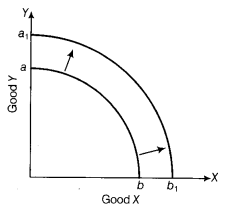PPC shift to a1 b1 as technology improves.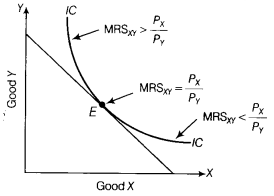Graph showing relation between MRS and $$\frac { { P }_{ X } }{ { P }_{ Y } }$$ at different points on indifference curve
The point where $${ MRS }_{ XY }=\frac { { P }_{ X } }{ { P }_{ Y } }$$ indicates the highest level of consumer’s satisfaction i.e. E is the point of maximum consumer satisfaction (or consumer equilibrium).
Or
The two conditions of consumer’s equilibrium are stated below:
(i) Ratio of Marginal Utility to Price of Two Goods are Equal to Marginal Utility of Money This condition can be expressed as $$\frac { M{ U }_{ X } }{ { P }_{ X } } =\frac { M{ U }_{ Y } }{ { P }_{ Y } } =M{ U }_{ M }$$
or $$\frac { M{ U }_{ X } }{ M{ U }_{ Y } } =\frac { { P }_{ X } }{ { P }_{ Y } }$$ [if MUX = 1]
where, MUX and MUY = Marginal Utilities of good X and good Y respectively
PX and PY = Price of good X and good Y respectively
MUM = Marginal utility of money
(ii) MU Falls as Consumption Increases If Marginal Utility (MU) does not fall as consumption increases, the consumer will end up buying only one good which is unrealistic and the consumer will never reach the equilibrium position.

The law of variable proportions shows the impact on output when units of variable factors are increased, keeping fixed factors constant in the short-run.

The law states that if more and more units of a variable factor are employed with fixed factors, Total Physical Product (TPP) increases at an increasing rate in the beginning, then increases at a diminishing rate and finally starts falling. In other words, as units of variable factors are increased, marginal product first increases, reaches its maximum point, starts to fall, and eventually becomes negative.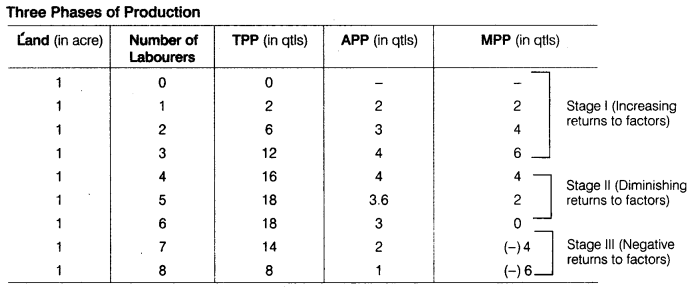Oligopoly is a form of market in which there are only few big sellers of a commodity and large number of buyers.
e.g. there are only few car producers in the Indian auto market such as Toyata, Ford, GM, Audi, BMW and Volkswagen.
Oligopoly market exhibits the features given below:

1. A Few Firms A few firms, large in size dominate the market for a commodity. Each firm commands a significant share of the market which can impact market price of the product.
2. Large Number of Buyers There are large number of buyers of a commodity. The number is so large that no individual buyer can impact market price of the product.
3. Entry Barriers There are various barriers to the entry of new firms. These barriers are almost similar to
those under monopoly. Entry of the new firm is extremely difficult, but not impossible.

Demand means the quantity of a commodity that consumers wish to purchase in the market in a given period of time and at various prices.
Factors affecting individual household demand are explained below:

1. Own Price of a Commodity When the price of a commodity rises in the market, its demand contracts and with a fall in price, its demand expands.
2. Price of Related Goods Demand for a commodity is also influenced by change in price of related goods.
These are of two types:

• Substitute Goods These are the goods which can be substituted for each other, such as tea and coffee or ball pen and ink pen. In case of such goods, increase in the price of one, causes increase in demand for the other and decrease in the price of one, causes decrease in the demand for the other.
• Complementary Goods These goods complete the demand for each other and are therefore, demanded together. Pen and ink, bread and butter, car and petrol, etc may be cited as examples. In case of complementary goods, a fall in the price of one, causes increase in the demand for the other and a rise in the price of one, causes decrease in the demand for the other.
3. Income of the Consumer Change in the income of the consumer also influences his demand for different goods. The demand for normal goods tends to increase with increase in income and vice-versa. On the other hand, the demand for inferior goods like coarse grain, etc. tends to decrease with increase in income and vice-versa.
4. Tastes and Preferences The demand for goods and services also depends on individual tastes and preferences. These terms are used in a broad sense. If there is a favourable change in tastes and preferences, demand increases and unfavourable change leads to decrease the demand.
5. Expectatibns If the consumer expects a significant change in the availability of the concerned
commodity in the near future, he may decide to change his present demand for the commodity. Particularly, if the consumer fears acute shortage of the commodity in the near future, he may raise his present demand for the commodity at its existing price.

(i) Yes, Marginal Revenue (MR) can be negative. It can be negative only when average revenue is decreasing or when the price is declining as under monopoly and monopolistic competition. This can be explained by the following example :
When price = ₹ 20, output = 50
Total revenue of 50 units (TR50) = P x Q
= 20 x 50 = ₹ 1,000
When price fall to ₹ 19, output =51
Total revenue of 51 units (TR51) = P x Q
= 19 x 51 = ₹ 969
MR51 =TR51 – TR50
= 969 – 1,000 = ₹ (31)
i.e., Marginal Revenue (MR) is negative.
(ii) TR = ₹ 15,000, P(=AR) = ₹ 150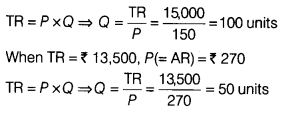Change in market demand = 50 – 100 = -50 units i.e. market demand falls by 50 units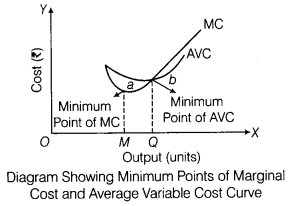1. False. because when Marginal Revenue (MR) is constant, Total Revenue (TR) is increasing at a constant rate, only when Marginal Revenue is zero, Total Revenue is constant.
2. False, Average Variable Cost (AVC) can fall even when Marginal Cost (MC) starts rising. See Average Variable Cost (AVC) and Marginal Cost (MC) corresponding to output range (MQ) in the diagram.
3. True, because in a situation of increasing returns to a factor, Marginal Product tends to rise accordingly, Total Product (TP) should be increasing at an increasing rate. Under diminishing returns to a factor, Marginal Product (MP) tends to fall. Falling Marginal Product (MP) implies that Total Product (TP) should be increasing, though at a decreasing rate.

In the short-run, equilibrium of a firm (under perfect competition) may occur when it is earning super normal profits or normal profits or is incurring super normal losses.
These conditions are discussed below :
(i) Super Normal Profit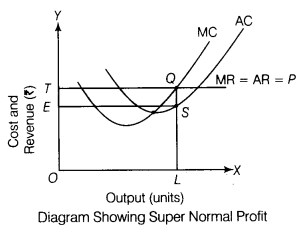In the given diagram, the producer is at equilibrium at point Q, where MR = MC, and MC cuts MR from below. At this point, Average Revenue (AR) exceed Average Cost (AC). So, the firm is earning abnormal profits equal to ESQT.
(ii) Super Normal Losses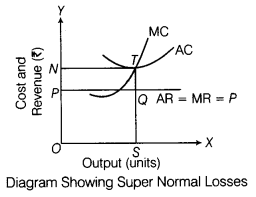In the given diagram, the firm is at equilibrium at point 0. At this point, AC exceeds AR, therefore the firm is incurring abnormal loss equal to NTQP.
(iii) Normal Profit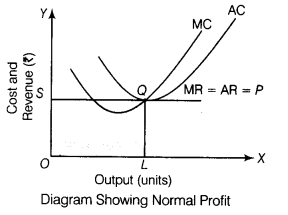In the given diagram, the firm is at equilibrium at point Q. At this point AR = AC. So, this depicts the condition of normal profits.
If the firm is incurring abnormal losses then it affects the economic value of sustainability.

Section – B

The government can borrow money from :
(i) The Reserve Bank of India
(ii) The rest of the world

Yes, I agree. The face value of currency notes is more than its materialistic value. These notes are accepted
by people because they are backed by the government of the country.

Public goods refers to the goods which cannot be provided by market mechanism due to its feature of non-rivalness and non-excludable in consumption, e.g. street light.

(d) Time deposits with banks

(i) At equilibrium, savings (S) are equal to planned investment (l). It is given that,
S = – 200 + Y
Equilibrium level of income is 2,000. Substituting the value of Y in the savings function, we get
S = -200 + 0.25 x 2,000
S =-200 + 500 ⇒ S =300
or l = 300 [v at equilibrium level of Income Savings (S) = Investment Expenditure (I)]
Thus, investment expenditure at equilibrium level of income is 300.
Autonomous consumption means the level of consumption expenditure when income is zero.
When Y = 0, Savings = -200
Consumption + Savings = Income Consumption + (-200) = 0
So, autonomous consumption = 200
Or
According to question, Marginal Propensity to consume (MPC) = $$\frac { 1 }{ 3 }$$ Marginal Propensity of Save (MPS)
We know that,
MPC + MPS = 1
∴    $$\frac { 1 }{ 3 }$$ MPS + MPS = 1
MPS = $$\frac { 3 }{ 4 }$$
∴ MPS = 0.75 and
MPC = 1 – 0.75 = 0.25 Consumption function (C) = $$\overline { C }$$ + bY,
where,
$$\overline { C }$$ = Autonomous Consumption = ₹ 100 crore
b = MPC = 025
So, consumption function (C) = 100 + 025 Y
Saving function (S) = $$-\overline { S }$$ + sY,
where,
$$-\overline { S }$$ = Dissavings = Autonomous consumption = 100 and
S = Marginal Propensity to Save = 0.75
Therefore, Savings Function (S) = -100 + 0.75Y

Difference between inflationary gap and deflationary gap are :

 Basis Inflationary Gap Deflationary Gap Meaning It is the excess of aggregate demand over and above its level required to maintain full employment equilibrium in the economy. It is the deficiency of aggregate demand required to maintain full employment equilibrium in the economy. Level of output Level of output is constant at full employment level of output is less than that at full employment. Major effect It leads to a situation of rise in general price level, without any rise in level of employment in economy. It leads to a situation where factors of production are not fully utilised, leading to unemployment in the economy.

1. Dividend received by an Indian from his investment in shares of a foreign company is included in National Income of India because it is a part of net factor income from abroad.
2. Money received by a family in India from relatives working abroad is not included in National Income of India because it is a kind of transfer income.
3. Interest received on loan given to a friend for purchasing a car is not included in National Income because loan is not being used for production purpose.
4. Dividend received by a foreigner from investment in shares of an Indian company is included in National
Income of India as a negative component, because it is a part of net factor income paid to rest of the world.

Money creation (or deposit creation or credit creation) by the banks is determined by

1. The amount of the initial fresh deposits and
2. Legal Reserve Ratio (LRR) i.e. the minimum ratio of deposit, legally required to be kept as cash by the banks. It is assumed that all the money that goes out of the banks, is re-deposited into the banks.

Let the LRR be 20% and there is a fresh deposit of ₹ 10,000. As required, the banks keep 20 %,i.e. ₹ 2,000 as cash. Suppose the banks lend the remaining ₹ 8,000. Those who borrow, use this money for making payments. As assumed, those who receive payments, put the money back into the banks. In this way, banks receive fresh deposits of ₹ 8,000. The banks again keep 20%, i.e. ₹ 1,600 as cash and lend ₹ 6,400, which is also 80% of the last deposits. The money again comes back to the banks leading to a fresh deposit of ₹ 6,400. The money goes on multiplying in this way and ultimately total money creation is ₹ 50,000.

Given the amount of fresh deposit and the LRR, the total money creation is
Total Money Creation = Initial Deposit x $$\frac { 1 }{ LRR }$$
Or
With the introduction of ‘Jan-Dhan Yojana’, many households have opened bank account. Due to this, primary deposits of commercial banks have increased. Banks create credit on the basis of primary deposits. Higher the primary deposits, higher is the credit creation capacity of comrftercial banks and vice-versa.

Now, with implementation of ‘Jan-Dhan Yojana’, since primary deposits have increased, reserves of bank to create credit have also swollen up. Therefore, credit creation capacity of commercial banks is increased manifolds.

Government budget is of immense help in fighting the inflationary and deflationary tendencies. To curb the inflationary tendency, the government can prepare a surplus budget. A surplus budget is one in which estimated receipts exceed the estimated expenses. Such a budget reduces the money supply in the economy. With a fall in the money supply, the purchasing power of people also fall, leading to a fall in the level of aggregate demand. As aggregate demand falls, the price level or the rate of inflation also falls.

To curb the deflationary tendency, the government can prepare a deficit budget. A deficit budget is one in which estimated expenses exceed the estimated receipts. Such a budget increases the money supply in the economy. With increase in money supply, the purchasing power of people also rise, leading to an increase in the level of aggregate demand. As aggregate demand rises, the price level also rises and rate of deflation begins to fall.

(i) Gross National Product at Market Price (GNPMP) by Income Method
Net Domestic Product at Factor Cost (NDPFC ) = Compensation of Employees + Operating Surplus (Interest + Rent + Profit) + Mixed Income
= ₹ 1,500 + (400 + 400 + 1,000) + 0
= ₹ 1,500 + 1800
= ₹ 3,300 crore
GNPMP = NDPFC + Net indirect Tax (Indirect tax – Subsidies) + Net Factor Income from Abroad (Factor income from abroad – Factor income to abroad) + Depreciation
= ₹ 3300 + (300 – 100) + (100 -150) + 150
= ₹ 3300 + 200 + (-50) + 150 = 3,300 + 300
= ₹ 3,600 crore

(ii) Gross National Product at Market Price (GNPMP) by Expenditure Method
Gross Domestic Product at Market Price (GDPMP) = Private Final Consumption Expenditure + Government Final Consumption Expenditure + Gross Domestic Capital Formation + Net Exports
= ₹ 2,200 + 850 + (500 + 150)+ (200 – 250)
= ₹ 2200 + 850 + 650 + (-50)
= ₹ 2,200 + 850 + 600 = ₹ 3650 crore
GNPMP = GDPMP + Net Factor Income from Abroad
= ₹ 3650 + (100 – 150) = 3,650 – 50 = ₹ 3,600 crore
Or
Steps for measuring National Income by Value Added Method (Product method) are enumerated below :
Step I Classify All the Production Units The units are traditionally classified into primary, secondary and tertiary sectors which are further classified into sub-sectors like agriculture, manufacturing, banking, etc.
Step II Estimate NVAFC of each industrial sector by taking the following sub-steps :

1. Estimate Value of Output It can be estimated by two ways :
• A sum of sales and net addition to stocks (If entire output is not sold within the year).
• Quantity of output multiplied by price (If entire output is sold within the year).
2. Deduct Intermediate Consumption It should be deducted from the value of output to arrive at GVAMO (Intermediate consumption already includes imports).
3. Deduct, Consumption of Fixed Capital It should be deducted from GVAMP to arrive at NVAMP.
4. Deduct Net Indirect Tax It should be deducted from NVAMP to arrive at NVAFC of an industrial sector.

Step III Take the sum of NVAFC of all the industrial sectors of the economy. By doing so, we will arrive at net
domestic product at factor cost.
ΣNVAFC = NDPFC
Step IV Add net factor income from abroad to NDPFC to arrive at National Income.
National Income (NNPFC) = NDPFC + Net Factor Income from Abroad (NFIA)
Precautions to be taken while computing National income by this method are given below :

1. Take the value of final product only i.e. intermediate goods not to be included.
2. Do not include exports, if total sales are given.

Multiplier It means the ratio of change in income to change in investment.
According to Keynes, “Investment multiplier tells us that when there is an increment of aggregate investment, income will increase by an amount, which is K times the increment of investment.”
Relationship between MPC and Multiplier (K)
The value of Multiplier is determined by the value of MPC.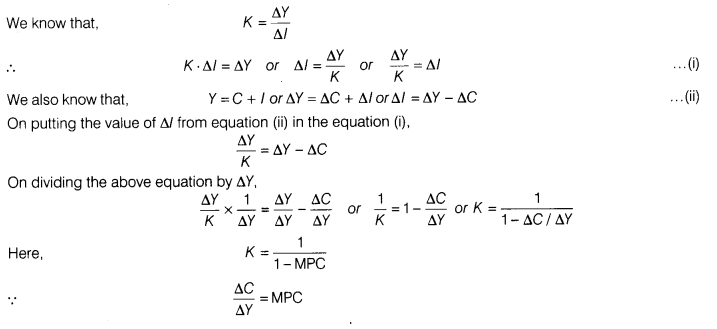This shows that there is a direct relationship between multiplier (K) and Marginal Propensity to Consume (MPC). As the value of MPC rises, the value of multiplier also rises and vice-versa.
e.g. if MPC = 0.75
then, $$\frac { 1 }{ 1-0.75 } =4$$
and when the value of MPC = 0.80, then K = $$\frac { 1 }{ 1-0.80 } =5$$
Thus, it is clear that the size of the Multiplier depends on the MPC. Higher the MPC, the larger the size of Multiplier and lower the MPC, the smaller is the size of Multiplier.